NEET  >  31 Year NEET Previous Year Questions: Chemical Kinetics - 1

# 31 Year NEET Previous Year Questions: Chemical Kinetics - 1

Test Description

## 17 Questions MCQ Test Chemistry Class 12 | 31 Year NEET Previous Year Questions: Chemical Kinetics - 1

31 Year NEET Previous Year Questions: Chemical Kinetics - 1 for NEET 2022 is part of Chemistry Class 12 preparation. The 31 Year NEET Previous Year Questions: Chemical Kinetics - 1 questions and answers have been prepared according to the NEET exam syllabus.The 31 Year NEET Previous Year Questions: Chemical Kinetics - 1 MCQs are made for NEET 2022 Exam. Find important definitions, questions, notes, meanings, examples, exercises, MCQs and online tests for 31 Year NEET Previous Year Questions: Chemical Kinetics - 1 below.
Solutions of 31 Year NEET Previous Year Questions: Chemical Kinetics - 1 questions in English are available as part of our Chemistry Class 12 for NEET & 31 Year NEET Previous Year Questions: Chemical Kinetics - 1 solutions in Hindi for Chemistry Class 12 course. Download more important topics, notes, lectures and mock test series for NEET Exam by signing up for free. Attempt 31 Year NEET Previous Year Questions: Chemical Kinetics - 1 | 17 questions in 35 minutes | Mock test for NEET preparation | Free important questions MCQ to study Chemistry Class 12 for NEET Exam | Download free PDF with solutions
 1 Crore+ students have signed up on EduRev. Have you?
31 Year NEET Previous Year Questions: Chemical Kinetics - 1 - Question 1

### Half life period of a first-order reaction is 1386 seconds. The specific rate constant of the reaction is: 

Detailed Solution for 31 Year NEET Previous Year Questions: Chemical Kinetics - 1 - Question 1

For a first order reaction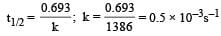31 Year NEET Previous Year Questions: Chemical Kinetics - 1 - Question 2

### For the reaction A + B →  products, it is observed that:  (1) On doubling the initial concentration of A only, the rate of reaction is also doubled and (2) On doubling the initial concentrations of both A and B, there is a change by a factor of 8 in the rate of the reaction. The rate of this reaction is given by:

Detailed Solution for 31 Year NEET Previous Year Questions: Chemical Kinetics - 1 - Question 2

When concentration A is doubled, rate is doubled. Hence order with respect to A is one.
When concentrations of both A and B are doubled, rate increases by 8 times hence order with respect to B is 2.
∴   rate = k [A]1 [B]2
Total order = 1 + 2 = 3

31 Year NEET Previous Year Questions: Chemical Kinetics - 1 - Question 3

### For the reaction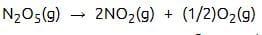the value of rate of disappearance of N2O5 is given as  6.25 × 10–3 mol L–1s–1. The rate of formation of NO2 and O2 is given respectively as : 

Detailed Solution for 31 Year NEET Previous Year Questions: Chemical Kinetics - 1 - Question 3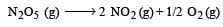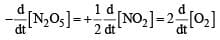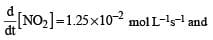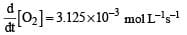31 Year NEET Previous Year Questions: Chemical Kinetics - 1 - Question 4

For an endothermic reaction, energy of activation is Ea and enthalpy of reaction of ΔH (both of these in kJ/mol). Minimum value of Ea will be. 

Detailed Solution for 31 Year NEET Previous Year Questions: Chemical Kinetics - 1 - Question 4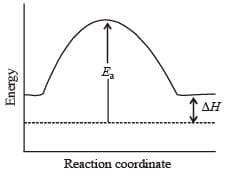Ea  >  ΔH

31 Year NEET Previous Year Questions: Chemical Kinetics - 1 - Question 5

During the kinetic study of the reaction, 2A + B → C + D, following results were obtained: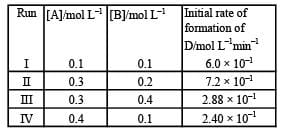Based on the above data which one of the following is correct? 

Detailed Solution for 31 Year NEET Previous Year Questions: Chemical Kinetics - 1 - Question 5

In case of (II) and (III) Keeping concentration of [A] constant, when the concentration of [B] is doubled, the rate quadruples. Hence it is second order with respect to B. In case of I & IV Keeping the concentration of [B] constant. when the concentration of [A] is increased four times, rate also increases four times. Hence, the order with respect to A is one. hence Rate = k [A][B]2

31 Year NEET Previous Year Questions: Chemical Kinetics - 1 - Question 6

The rate of the reaction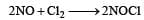is given by the rate equation rate = k [NO]2 [Cl2]      The value of the rate constant can be increased by:

Detailed Solution for 31 Year NEET Previous Year Questions: Chemical Kinetics - 1 - Question 6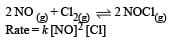The value of rate constant can be increased by increasing the temperature.
∴ Correct choice : (b)

31 Year NEET Previous Year Questions: Chemical Kinetics - 1 - Question 7

Which one of the following statements for the order of a reaction is incorrect ? 

Detailed Solution for 31 Year NEET Previous Year Questions: Chemical Kinetics - 1 - Question 7

order of reaction may be zero, whole number or fractional.

31 Year NEET Previous Year Questions: Chemical Kinetics - 1 - Question 8

For the reaction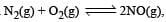, the equilibrium constant is K1. The equilibrium constant is K2 for the reaction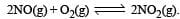.What is K for the reaction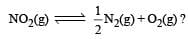Detailed Solution for 31 Year NEET Previous Year Questions: Chemical Kinetics - 1 - Question 8

For the reaction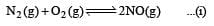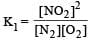For the reaction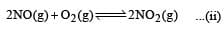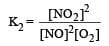For the reaction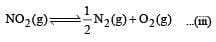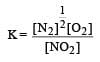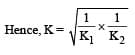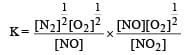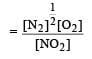31 Year NEET Previous Year Questions: Chemical Kinetics - 1 - Question 9

The rate of the reaction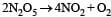can be written in three ways :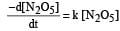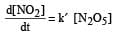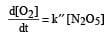The relationship between k and k' and between k and k"are :

[2011 M]

Detailed Solution for 31 Year NEET Previous Year Questions: Chemical Kinetics - 1 - Question 9

Rate of disappearance of reactants = Rate of appearance of products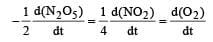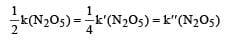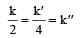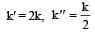31 Year NEET Previous Year Questions: Chemical Kinetics - 1 - Question 10

The unit of rate constant for a zero order reaction is [2011 M]

Detailed Solution for 31 Year NEET Previous Year Questions: Chemical Kinetics - 1 - Question 10

Rate = K(A)0 Unit of K = mol L–1 sec–1

31 Year NEET Previous Year Questions: Chemical Kinetics - 1 - Question 11

In a reaction, A + B → Product, rate is doubled when the concentration of B is doubled, and rate increases by a factor of 8 when the concentrations of both the reactants (A and B) are doubled rate, law for the reaction can be written as : 

Detailed Solution for 31 Year NEET Previous Year Questions: Chemical Kinetics - 1 - Question 11

According to statement given in the question, it is clear that r ∝ [A]2 and r ∝ [B]
That means order of reaction with respect to B is 1 and w.r.t A is 2
Hence, Rate = k[A]2[B]1

31 Year NEET Previous Year Questions: Chemical Kinetics - 1 - Question 12

In a zero-order reaction for every 10° rise of temperature, the rate is doubled. If the temperature is increased from 10°C to 100°C, the rate of the reaction will become : 

Detailed Solution for 31 Year NEET Previous Year Questions: Chemical Kinetics - 1 - Question 12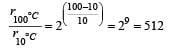*Multiple options can be correct
31 Year NEET Previous Year Questions: Chemical Kinetics - 1 - Question 13

Activation energy (Ea) and rate constants (k1 and k2) of a chemical reaction at two different temperatures (T1 and T2) are related by : [2012 M]

Detailed Solution for 31 Year NEET Previous Year Questions: Chemical Kinetics - 1 - Question 13

According to Arrhenius equation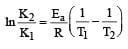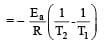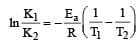31 Year NEET Previous Year Questions: Chemical Kinetics - 1 - Question 14

What is the activation energy for a reaction if its rate doubles when the temperature is raised from 20°C to 35°C? (R = 8.314 J mol–1 K–1) [NEET 2013]

Detailed Solution for 31 Year NEET Previous Year Questions: Chemical Kinetics - 1 - Question 14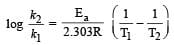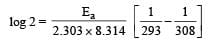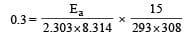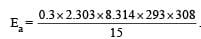= 34673 J mole-1
= 34.7 J mole-1

31 Year NEET Previous Year Questions: Chemical Kinetics - 1 - Question 15

A reaction having equal energies of activation for forward and reverse reaction has : [NEET 2013]

Detailed Solution for 31 Year NEET Previous Year Questions: Chemical Kinetics - 1 - Question 15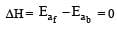31 Year NEET Previous Year Questions: Chemical Kinetics - 1 - Question 16

A reaction is 50% completed in 2 hours and 75% completed in 4 hours. The order of reaction is     [NEET Kar. 2013]

Detailed Solution for 31 Year NEET Previous Year Questions: Chemical Kinetics - 1 - Question 16

For a first order reaction, t75% = 2 × t50%

31 Year NEET Previous Year Questions: Chemical Kinetics - 1 - Question 17

For a reaction between A and B the order with respect to A is 2 and the order with respect to B is 3. The concentrations of both A and B are doubled, the rate will increase by a factor of: [NEET Kar. 2013]

Detailed Solution for 31 Year NEET Previous Year Questions: Chemical Kinetics - 1 - Question 17

Rate = k[A]2[B]3 when concentrations of both A and B are doubled then rate = k[2A]2[2B]3 = 32 k[A]2[B]3
∴       rate will increase by a factor of 32.

## Chemistry Class 12

157 videos|385 docs|261 tests
 Use Code STAYHOME200 and get INR 200 additional OFF Use Coupon Code
Information about 31 Year NEET Previous Year Questions: Chemical Kinetics - 1 Page
In this test you can find the Exam questions for 31 Year NEET Previous Year Questions: Chemical Kinetics - 1 solved & explained in the simplest way possible. Besides giving Questions and answers for 31 Year NEET Previous Year Questions: Chemical Kinetics - 1, EduRev gives you an ample number of Online tests for practice

## Chemistry Class 12

157 videos|385 docs|261 tests

### How to Prepare for NEET

Read our guide to prepare for NEET which is created by Toppers & the best Teachers